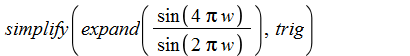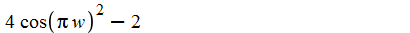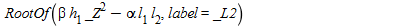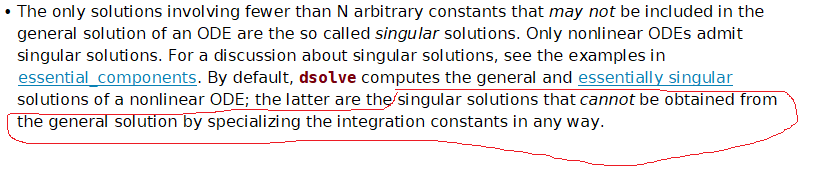## why order of coeffs not respected...

unprotect(D); f := proc (x, y) options operator, arrow; (295849/5841396)*x^2-(29441/324522)*y*x+(33995/216348)*y^2-(5989/14751)*x+(3635/4917)*y+1 end proc; 295849 2 29441 33995 2 5989 f := (x, y) -> ------- x - ------ y x + ------ y - ----- x 5841396 324522 216348 14751 3635 + ---- y + 1 4917 coeffs(f(x, y)); -5989 3635 295849 33995 -29441 1, -----, ----, -------, ------, ------ 14751 4917 5841396 216348 324522 A, B, C, D, E, F := %; -5989 295849 33995 -29441 3635 A, B, C, D, E, F := 1, -----, -------, ------, ------, ---- 14751 5841396 216348 324522 4917

## Recursive Assignment Errors...

Is there any way we can get maple to tell us which variable we are recursively assigning?

## How to simplify sin(4*pi*w)/sin(2*pi*w) (Answer:...

I would like to simplify

sin(4*pi*w)/sin(2*pi*w)

The answer I am looking for is 2*cos(2*pi*w).

Does anyone have an idea how to do this is Maple? Some earlier version of Maple I used could do this,

simplify( sin(4*pi*w)/sin(2*pi*w) ) =  2*cos(2*pi*w)

There a plenty of different trigonometric representations for the answer, so my hypothesis is that I should somehow tell to Maple which I am looking for.

For exampleGivesWhich is better, but still not the answer I am looking for

Best Regards,

P

## how to solve problem...

RootOf(_Z^2*beta*h-alpha*l*l, label = _L2)## Derivative with numerical method...

 >>>>>(1)
 >(2)
 >(3)

Using numerical methods, I cannot calculate the derivative of sd with respect to r at r = 2 and t = 4.

Oliveira.

 >## Dirichlet conditions and Neumann values...

Does anyone know how to enter in the pdsolve function Dirichlet conditions and Neumann values?

Oliveira.

## how to display the time in standard format...

I am reading the help page for "time" and it says that it will display the time elapsed since the maple kernel was loaded, but I just want output that is the value of the time and date displayed on my computer at the point the input in the command line is exrcuted. How do I do this?

## Combining solve and plot commands...

Hi,

I am struggling with one task involving solve and plot commands. I would appreciate if I could get some help. What I need to do is as follows:

1. Define alpha between 0 and 1.

2. Solve an equation f(x,alpha) =0 for x by taking different values of alpha.

3. Of all values of x obtained in step 2, choose the value of x between 0 and 1 and discard all others. I have technically shown that there will be exactly one value that is acceptable and all other values of x can be discarded.

4. For different values of alpha and correspondingly selected values of x in step 3, plot and display function g(x,alpha) with function value on the vertical axis and alpha on the horizontal axis.

Regards,

Omkar

## Why is this an error?...

solve({-infinity < a , a < -1, -1 < b ,  b < 0});

## Seeking for a question about a bouncing ball....

Hi,

does anyone remember of a recent question (maybe a post) about a bouncing ball over a hilly ground?
I can't put the finger on it.

TIA

## Why is this piecewise plot giving an error? Pls he...

f(x) := piecewise(0 < x, x^(3/2)*sin(1/x), x = 0, 0, undefined);
plot(f(x));

gives me the following error:

Error, (in plot) incorrect first argument piecewise(0 < x, (HFloat(2.739493386336394e-116)+HFloat(2.739493386336394e-116)*I)*x^(3/2), x = 0, 0, undefined)

I just want to see the function plot. With Wolfram Alpha this is no deal at all!

## How do I plot a function under some constrainets...I used the implicit function to draw two images, how to display only the intersection of two images? Or, how do I draw the x^2+y^2+z^2=1 image under x+y+z=0 condition? Code show as above.Thank you.

## use of subs with the "``" symbols...

Is it because I have handed subs a multiset or is it because I used the "``" things in the code producing my set? I seemed to remember algsubs and subs working perfectly well regardless of the dimensions of the argument in previous times i have needed it.

 >(1)
 >>## dsolve returns signular solution which can be obta...

Why Maple returns -1/x as singular solution below when this solution can be obtained from the general solution when constant of integration is zero?

 > restart;
 > ode:=2*y(x)+2*x*y(x)^2+(2*x+2*x^2*y(x))*diff(y(x),x) = 0; dsolve(ode,singsol=false);> sol:=[dsolve(ode,singsol=essential)];> subs(_C1=0,sol)>